# H-Vectorial Modal Analysis for Anisotropic Waveguide

Compatibility:

Assuming ∂ ⁄ ∂z = 0 and regarding n0  as an effective refractive index, Equation 23 is reduced to a basic equation for the guided-mode analysis of anisotropic optical waveguides. Hence, from (9) we get the following Helmholtz equation: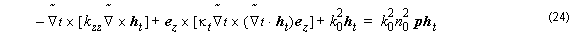One can cast the Helmholtz equation into the following matrix form: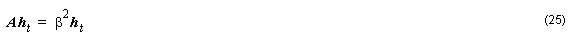where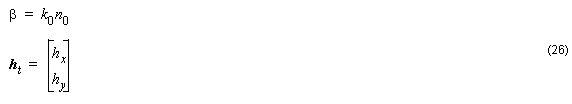and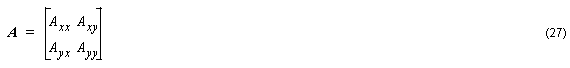The differential operators are defined as: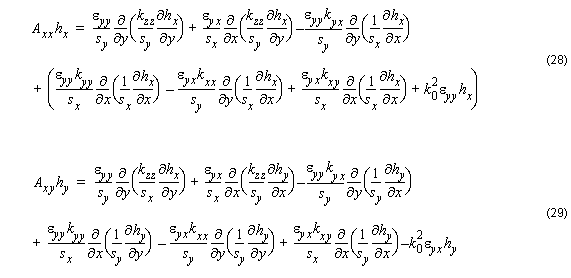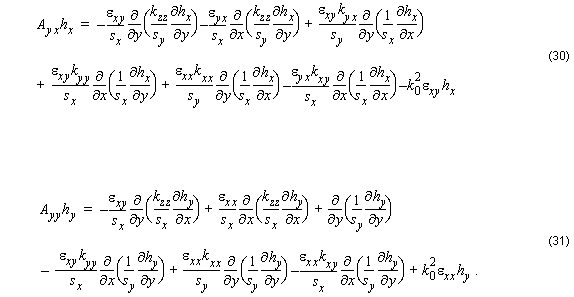It is noted that in Equations  – , both the material and the geometrical properties of the waveguides contribute to the polarization dependence,

Axx Ayy , and coupling:

Axy ≠ 0 and Ayx ≠ 0 .

Like in E-Formulation Equation 25 can be solved by an efficient Arnodi method that takes into account the sparsity under consideration and computes just a few number of eigenvalues and eigenvectors that represent the propagation constants and fields distributions for the wanted modes.